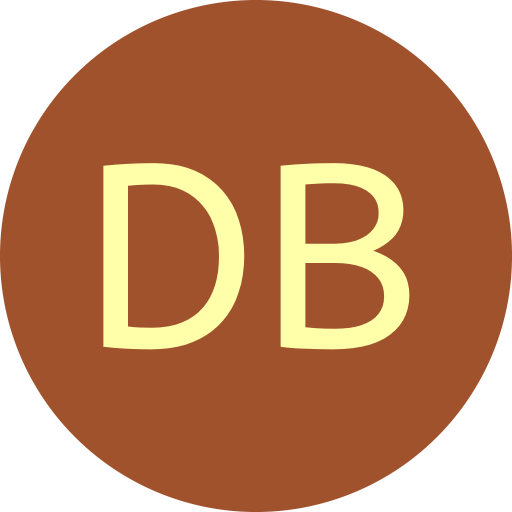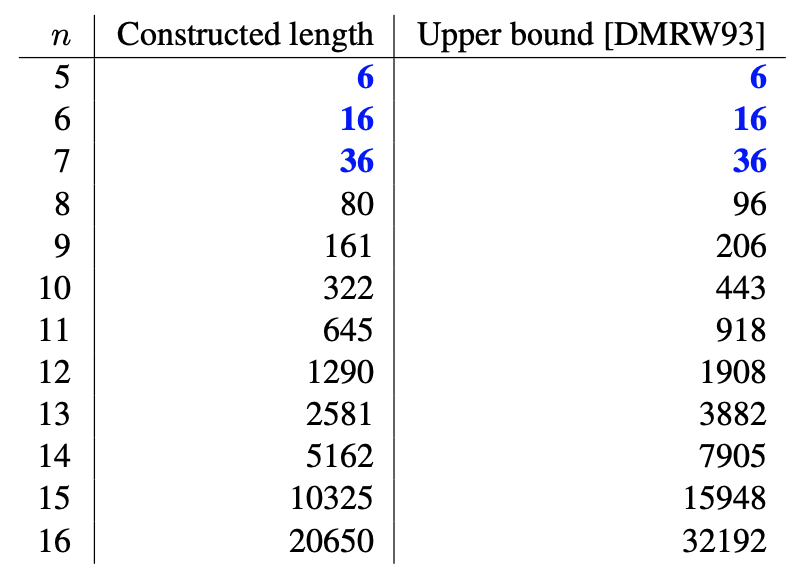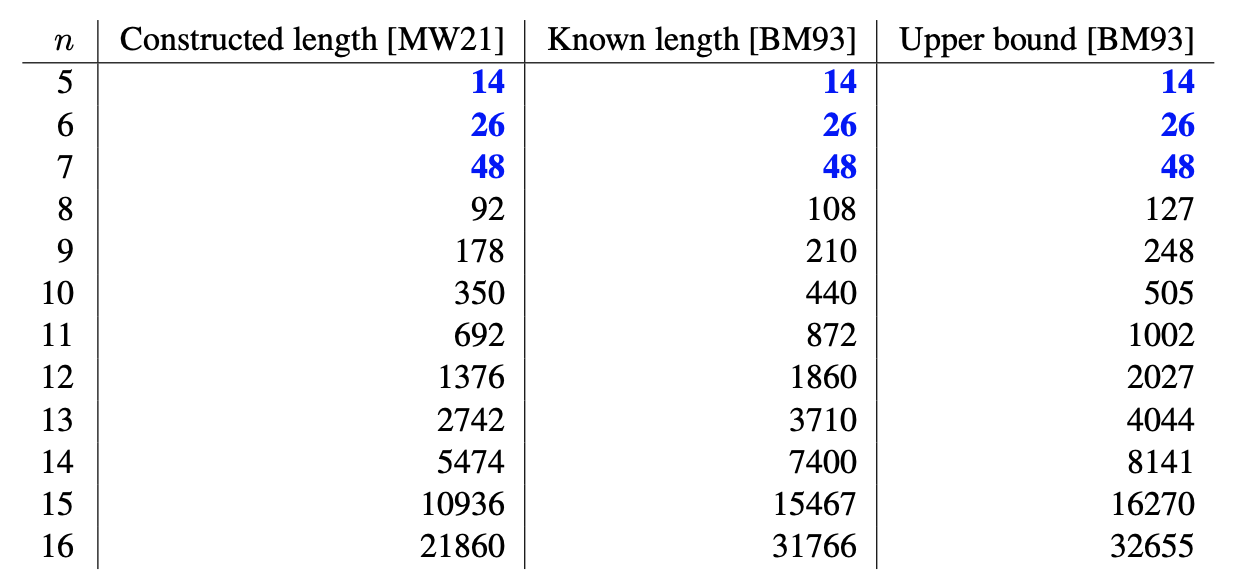#De Bruijn Sequence and Universal Cycle Constructions

Recursive Construction Output
 Type Orientable sequence Aperiodic orientable sequence Order $n$ 2 3 4 5 6 7 8 9 10 11 12 13 14 15 16 17 18 19 20 21 22 23 24 25
Orientable sequences

An orientable sequence or orientable universal cycle is a UC of order $n$ for a set $\mathbf{S}$ that does not contain both a string and its reversal, i.e., if $\mathbf{S}$ contains $w$ then it does not contain its reversal $w^R$ [DMRW93,MW21]. Thus $\mathbf{S}$ necessarily excludes palindromes.

Orientable UCs do not exist for $n<5$, and 001101 is a longest orientable UC for $n=5$. Lempel's lift $\mathcal{L}$ can be applied to recursively construct orientable UCs with length at least 63% of the trivial upper bound $2^{n-1} - 2^{\lfloor (n-1)/2\rfloor}$ [MW21]. Tighter bounds are known as given in the table below [DMRW93].

Recursive orientable UC construction

Let $\mathcal{O}_n$ denote an orientable UC of order $n$ and length $m$ with odd weight and the following property: it has exactly one substring $0^{n-4}$. Let $\mathcal{O}_{n+1}$ denote the string in $\mathcal{L}(\mathcal{O_n})$ replacing the unique substring $1^{n+1-4}$ with $1^{n+1-3}$ when its weight is even. The resulting orientable UC has length $2m$ or $2m{+}1$, has odd weight, and has the property of having exactly one substring $0^{n+1-4}$.

Example

Let $\mathcal{O}_6 = \underline{00}1010111$. Then applying $\mathcal{L}$ yields $\mathcal{O}_7 = \underline{000}110010111001101$ which has odd weight. Applying $\mathcal{L}$ again yields 000010001101000100111101110010111011 which has even weight so the unique substring 1111 is replaced with 11111 to yield $$\mathcal{O}_8 = \underline{0000}100011010001001111101110010111011.$$

The implementation used above applies an orientable sequence of order $n=8$ of length 80 as a base case for recursively computing larger orders. Brute force found optimal length orientable sequences for $n<8$. Below is a table of the length of orientable sequences constructed compared to the upper bound from [DMRW93] or from exhaustive search.#### Aperiodic orientable sequences

The linear relative of orientable UCs are known as aperiodic orientable sequences (or aperiodic 2-orientable window sequences in [BM93]). Trivially, they can be obtained from an orientable UC by copying the length $n{-}1$ prefix and appending it to the end, and a trivial upper bound for their maximal length is $2^{n-1} - 2^{\lfloor (n-1)/2\rfloor} + (n{-}1)$. Longer constructions that have length at least 2/3 optimal can also be constructed by applying the properties of the Lempel's lift [MW21].

Recursive aperiodic orientable sequence construction

Let $\mathcal{A}_n$ denote an aperiodic orientable sequence of order $n$ and length $m$ with the following property: it begins with $0^{n-1}$ and ends with $1^{n-1}$. Let $\mathcal{L}(\mathcal{A_n}) = \{U,\overline{U} \}$, where $U$ begins with 0. Construct $\mathcal{A}_{n+1}$ by joining $U$ with the reversal of $\overline{U}$ overlapping the alternating suffix of the former with the alternating prefix of the latter. When $n$ is even, the overlap has length $n$; when $n$ is odd, the overlap has length $n{-}1$. $\mathcal{A}_{n+1}$ retains the property of beginning with $0^n$ and ending with $1^n$.

Example

Let $\mathcal{A}_3 = 0011$. $\mathcal{L}(0011) = \{00010, 11101\}$ and $\mathcal{A}_4 = 000\underline{10}111$, where the overlap is underlined. Continuing, $\mathcal{L}(00010111) = \{000011010, 111100101\}$ and $\mathcal{A}_5 = 00001\underline{1010}01111$.

Below is a table of the length of aperiodic orientable sequences obtained from this construction compared to sequence lengths found in [BM93] and the trivial upper bound.(Aperiodic) orientable sequences have application in robotic position-location systems allowing a robot to determine both its location and orientation.